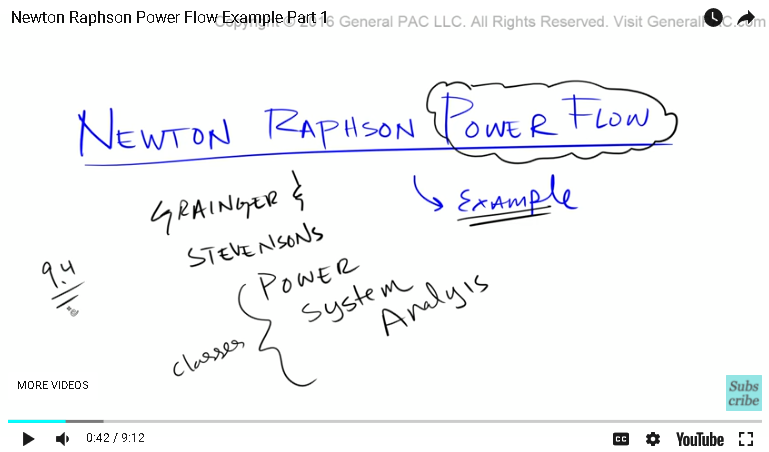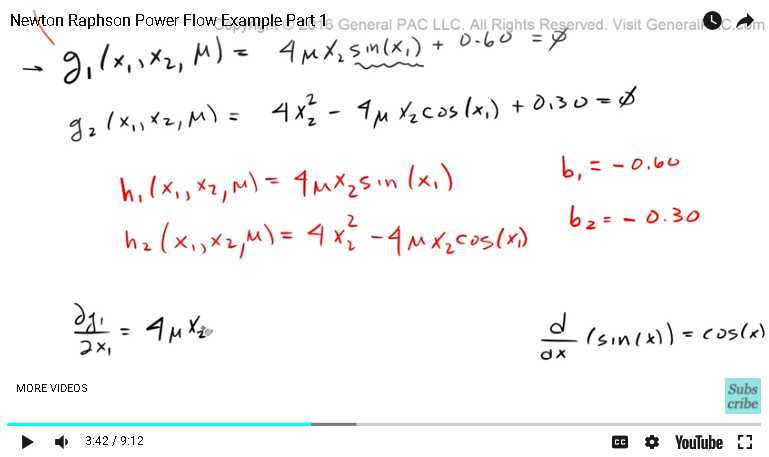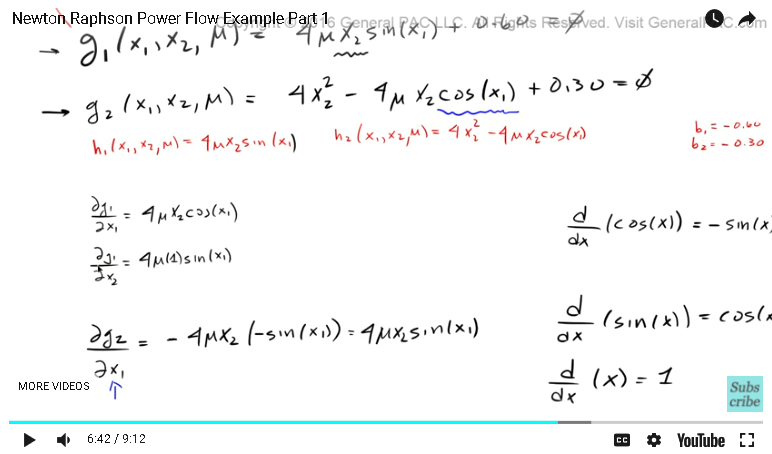This video was brought to you by GeneralPAC.com, making power systems Intuitive, Open and Free for Everyone, Everywhere. Consider subscribing and supporting through patreon.com/GeneralPAC. This is a mechanism for you to support us financially so we can continue making high quality power system video tutorials. Our corporate sponsor for this topic is AllumiaX.com from Seattle, Washington. Contact them for industrial and commercial power system studies.

The Newton-Raphson Power Flow Example.

In this tutorial, we'll be doing a practical example on power flow but using the Newton-Raphson method. This is more of an example-based tutorial rather than going through what the theory says and how the theory works. This is just an example-based tutorial.

Okay, this example really comes from Granger and Stevenson's Power System Analysis book. This book is used in many different classes and universities, but we're going to be going over example 9.4 within this book. If you want to follow along its section 9.3 and example 9.4.Okay, so like I said, this is going to be an example-based tutorial and the first equation that's presented is G1 of X1 is equal to H1, UG2. Initially, these are the two equations that are presented in example 9.4 of the book, that is about here, and then with these equations we're also presented with G1 is equal to four times U, two times sin of X1 equal to zero and G2 squared X2 that is equal to zero.

So, we're provided with these two equations. The first thing to know, notice that this equation and these two equations are the same thing, right? Which means H1 of X1, X2 and U, that is equal to 4U, X2, sin of X1, okay? H2 of X1, X2 U is equal 4 x 2 squared minus 4U, X2 cosine of X1. So H1 and H2 are defined by these three equations, which means that B1 is equal to -0.60. Because B1 is already negative here and we have a positive there. So B1 then has to be a negative for that to be positive. Then B2 is also -0.30. So the next part that we're presented to, and this is the part that really confuses a lot of people, is the actual partial differential equation.

So now what we're going to say is that the partial derivative of DG1 with respect to DX1. Now remember, DG1 is this right here. So now we have to take this equation and then do a partial derivative with respect to the X1 variable. So now it will become this. 4 x U, U stays where it is because we're multiplying, X2 stays where it is because X2 has nothing to do with X1 but guess what. There's a sin term right here and the derivative of sin of X, if we all remember, is equal to the cosine of X. So the derivative of sin is equal to the cosine. So because we're taking the derivative of G1 with respect to X1 we only take the derivative of this term here, which is equal to cosine of X1. Then this right here, because it's a constant, if we take the derivative of a constant that will equal 0. So here's our first equation.So now we have to take the partial derivative of DG1 with respect to DX2. So we're taking the same equation here but now we have to take the derivative with respect to DX2. Keep in mind that DX2 that DX2 is this term right there. So, that's the only term that is going to be really affected by the partial derivative. Also, keep in mind that the derivative of the X term, if it's just the derivative of X, well that is equal to just simply 1, which means that if we take the derivative of this term right here with respect to X2 and then that will equal 4 x U x 1, the derivative of X2 is just 1. Times that by the sin of X1. So remember the constant term just goes to 0.

So now, I'll clear the screen up a little bit so it's easier for us to work in. So the next thing is that we have to take the partial derivative of DG2 with respect to the X1 term and DG2 is this equation here and remember we are only concerned about DX1 at this point. So DX1 as you can see is this cosine term. There's no other X1 term in this equation. Well the first term goes to zero because there's no X1 term there. This third term, which is a constant, that's also going to go to zero because there's no X1 there so the only thing that's left is this term right here. If you remember, the derivative of cosine of X, that's going to equal - sin of X. So the derivative of cosine is going to equal -sin.

Our equation is going to be -4 x U, U stays where it is because it's part of this group, X2 stays where it is because we're only concerned about this term right here, and that is going to equal -sin of X1. Remember this term goes to 0. These two negatives obviously cancel out so that becomes just the positive 4UX2 sin of X1. So now I'm going to move this here. Now we're going to take the partial derivative of DG2 with respect to the X2 term. Again, we're here but now we are only concerned about the X2 term. So X2 is right there. There is one X2 there and there's one X2 there. So everything else we don't care about any other term. So now that is going to equal ... Well the derivative of X2, if you take the derivative of that, then we know that the 2 comes out of the coefficient and we subtract 1 from 2 then it becomes 2X. We all know that the derivative of X is just equal to 1.So when we take a partial derivative of this term here with respect to X2 that's going to become 8X2 and we subtract this term right here. Now, remember the derivative of just X2 term by itself is just equal to 1. So that is going to equal 4 x U x 1 x cosine of X1. There you have it. So this is now our fundamental building block of our Jacobi matrix, which we'll get into in a little bit. So now that we've covered kind of the introduction and, like I said this is more of an example-based introduction of the Newton-Raphson method and we'll really build on top of this example but I don't want to make this video too long so we'll conclude part one here.

As always, if you haven't subscribed yet please do so by clicking on the link that's on the bottom right corner of the screen "Please subscribe to this channel." Now if you have any questions there should be a link at the bottom of this video to the Q&A forum and the Q&A forum is hosted by the generalpac.com website so if you have any questions go ahead and just go to that forum and ask away.

Now in part two we'll just continue where we left off. Thank you. This video was brought to you by generalpac.com Making Power Systems Intuitive.

#### Greetings from the GeneralPAC Team!

We make high-quality Power Systems Video Tutorials on complex topics that are free and open to everyone!  Thank you so much for supporting us through Patreon so we can continue doing good and valuable work.

What is Patreon and why do we use it?

Patreon is a fantastic portal that allows our fans and community to make monthly contribution (like Netflix subscription) so we can continue creating high-quality power systems video tutorials. In return, you get access to incredible perks like voting on future topics, getting your questions answered, access to VIP Q/A webinars with the creators of GeneralPAC, and much more! We THANK YOU for supporting us

Why do we need your support?

An incredible amount of time and effort is needed to develop high-quality video tutorials. Each video (Part 1 for example) takes approximately 10 hours to complete which includes learning the concept ourselves, brainstorming creative ways to teach and explain the concepts, writing the script, audio recording, video recording, and editing. It's no wonder why Hundreds-of-Thousands of people have watched, liked, subscribed, and left positive comments on Youtube channel. Your support truly makes all the difference.